# RD Sharma Solutions for Class 6 Maths Chapter 1: Knowing Our Numbers Exercise 1.1

## RD Sharma Solutions for Class 6 Maths Exercise 1.1 PDF

The main objective of providing exercise wise solutions in PDF is to help students speed up their exam preparation. Understanding the concepts before solving the exercise wise problems is very important to obtain a better score in exam.The solutions are designed based on the latest CBSE syllabus and exam pattern. These solutions guide students to study well and solve lengthy problems in a shorter duration. The students can download PDF of solutions which are available in PDF format easily. RD Sharma Solutions for Class 6 Maths Chapter 1 Knowing Our Numbers Exercise 1.1 are provided here.

## RD Sharma Solutions for Class 6 Maths Chapter 1: Knowing Our Numbers Exercise 1.1 Download PDF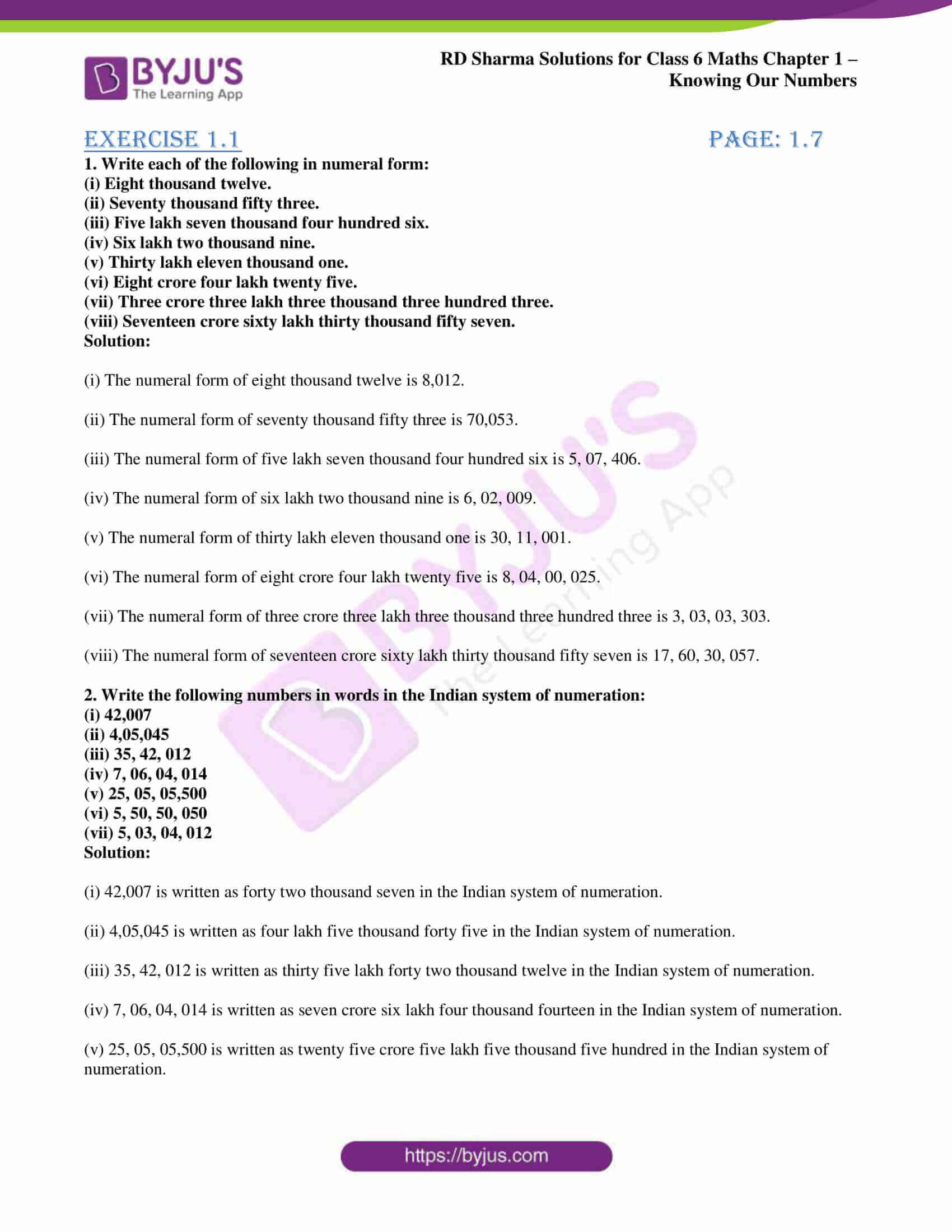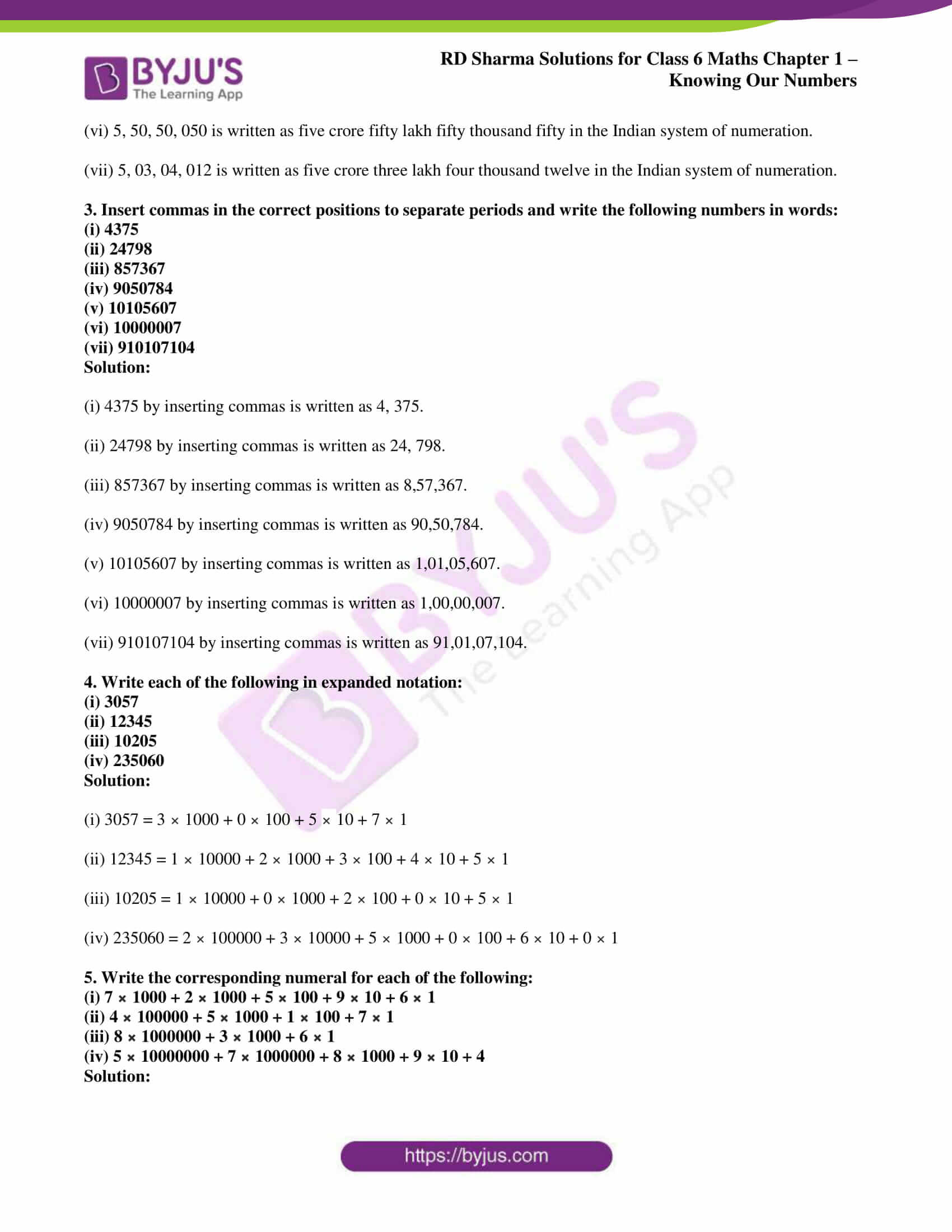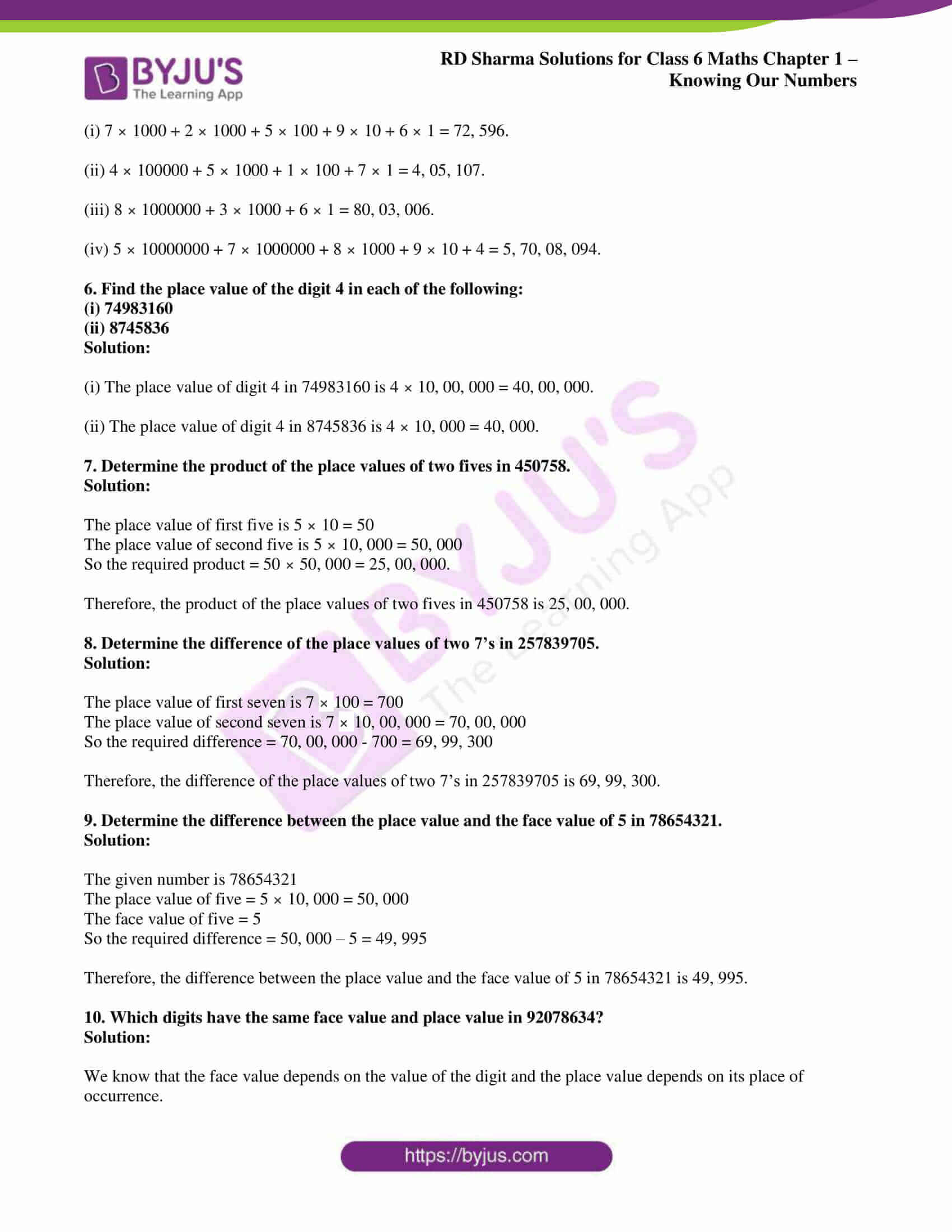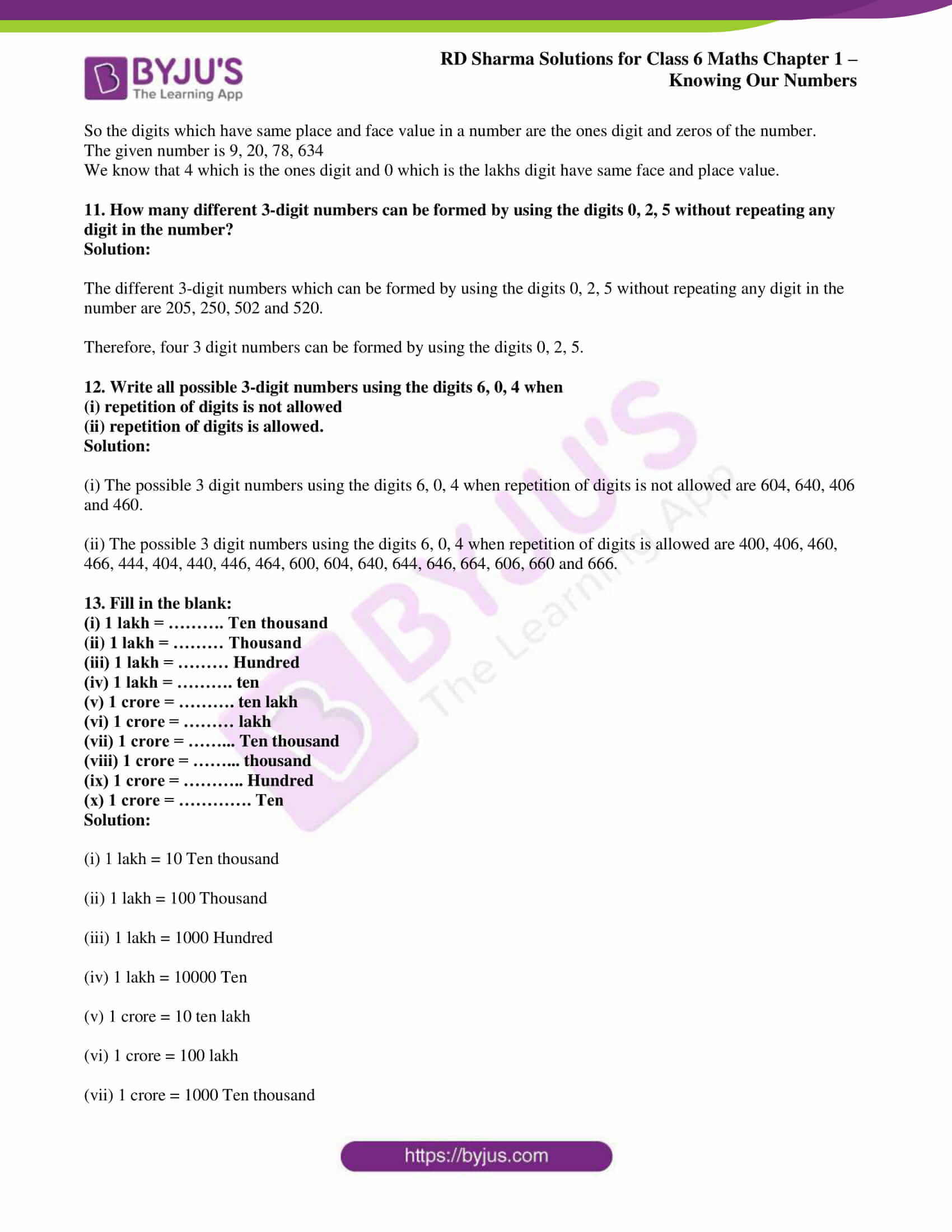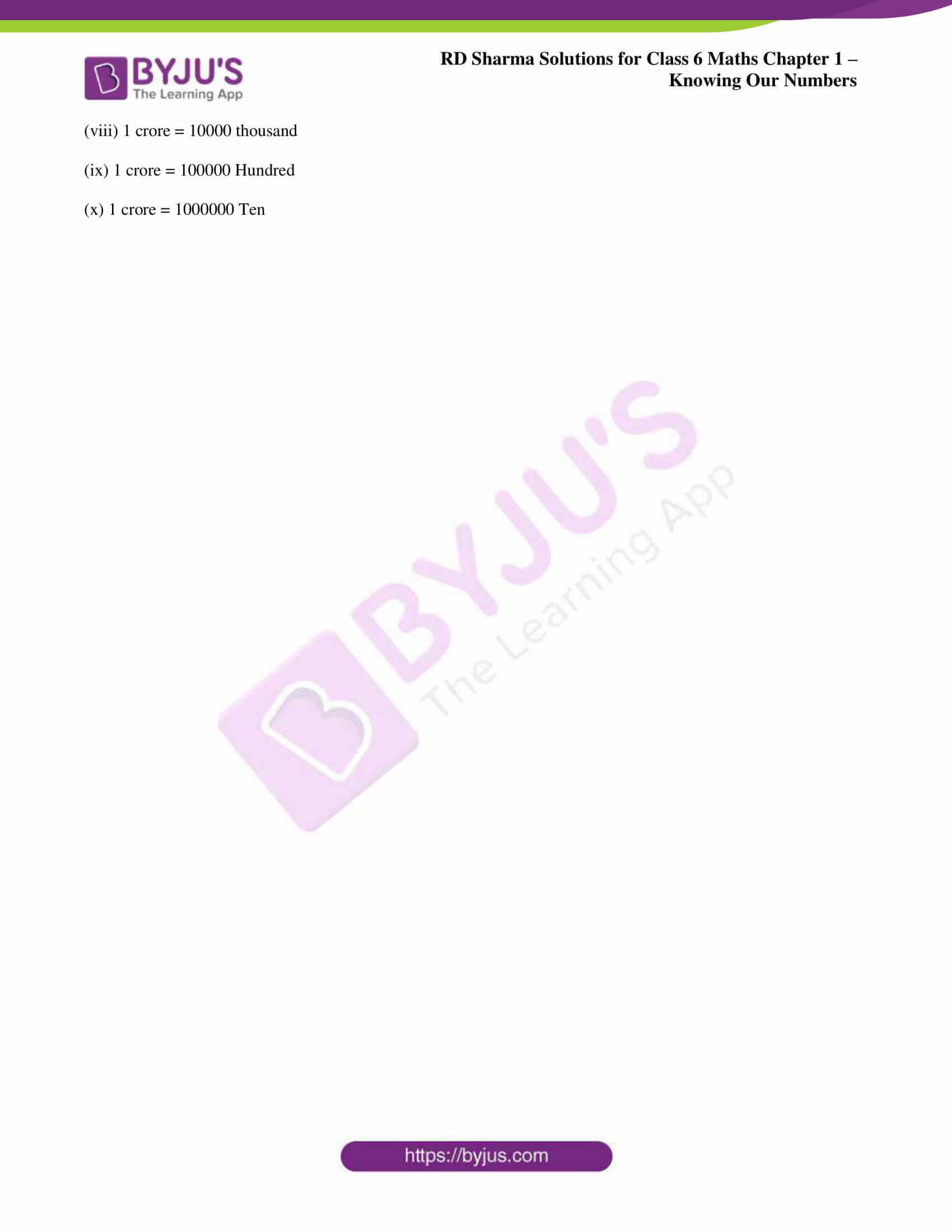### Access answers to Maths RD Sharma Solutions for Class 6 Chapter 1: Knowing Our Numbers Exercise 1.1

1. Write each of the following in numeral form:

(i) Eight thousand twelve.

(ii) Seventy thousand fifty three.

(iii) Five lakh seven thousand four hundred six.

(iv) Six lakh two thousand nine.

(v) Thirty lakh eleven thousand one.

(vi) Eight crore four lakh twenty five.

(vii) Three crore three lakh three thousand three hundred three.

(viii) Seventeen crore sixty lakh thirty thousand fifty seven.

Solution:

(i) The numeral form of eight thousand twelve is 8,012.

(ii) The numeral form of seventy thousand fifty three is 70,053.

(iii) The numeral form of five lakh seven thousand four hundred six is 5, 07, 406.

(iv) The numeral form of six lakh two thousand nine is 6, 02, 009.

(v) The numeral form of thirty lakh eleven thousand one is 30, 11, 001.

(vi) The numeral form of eight crore four lakh twenty five is 8, 04, 00, 025.

(vii) The numeral form of three crore three lakh three thousand three hundred three is 3, 03, 03, 303.

(viii) The numeral form of seventeen crore sixty lakh thirty thousand fifty seven is 17, 60, 30, 057.

2. Write the following numbers in words in the Indian system of numeration:

(i) 42,007

(ii) 4,05,045

(iii) 35, 42, 012

(iv) 7, 06, 04, 014

(v) 25, 05, 05,500

(vi) 5, 50, 50, 050

(vii) 5, 03, 04, 012

Solution:

(i) 42,007 is written as forty two thousand seven in the Indian system of numeration.

(ii) 4,05,045 is written as four lakh five thousand forty five in the Indian system of numeration.

(iii) 35, 42, 012 is written as thirty five lakh forty two thousand twelve in the Indian system of numeration.

(iv) 7, 06, 04, 014 is written as seven crore six lakh four thousand fourteen in the Indian system of numeration.

(v) 25, 05, 05,500 is written as twenty five crore five lakh five thousand five hundred in the Indian system of numeration.

(vi) 5, 50, 50, 050 is written as five crore fifty lakh fifty thousand fifty in the Indian system of numeration.

(vii) 5, 03, 04, 012 is written as five crore three lakh four thousand twelve in the Indian system of numeration.

3. Insert commas in the correct positions to separate periods and write the following numbers in words:

(i) 4375

(ii) 24798

(iii) 857367

(iv) 9050784

(v) 10105607

(vi) 10000007

(vii) 910107104

Solution:

(i) 4375 by inserting commas is written as 4, 375.

(ii) 24798 by inserting commas is written as 24, 798.

(iii) 857367 by inserting commas is written as 8,57,367.

(iv) 9050784 by inserting commas is written as 90,50,784.

(v) 10105607 by inserting commas is written as 1,01,05,607.

(vi) 10000007 by inserting commas is written as 1,00,00,007.

(vii) 910107104 by inserting commas is written as 91,01,07,104.

4. Write each of the following in expanded notation:

(i) 3057

(ii) 12345

(iii) 10205

(iv) 235060

Solution:

(i) 3057 = 3 × 1000 + 0 × 100 + 5 × 10 + 7 × 1

(ii) 12345 = 1 × 10000 + 2 × 1000 + 3 × 100 + 4 × 10 + 5 × 1

(iii) 10205 = 1 × 10000 + 0 × 1000 + 2 × 100 + 0 × 10 + 5 × 1

(iv) 235060 = 2 × 100000 + 3 × 10000 + 5 × 1000 + 0 × 100 + 6 × 10 + 0 × 1

5. Write the corresponding numeral for each of the following:

(i) 7 × 1000 + 2 × 1000 + 5 × 100 + 9 × 10 + 6 × 1

(ii) 4 × 100000 + 5 × 1000 + 1 × 100 + 7 × 1

(iii) 8 × 1000000 + 3 × 1000 + 6 × 1

(iv) 5 × 10000000 + 7 × 1000000 + 8 × 1000 + 9 × 10 + 4

Solution:

(i) 7 × 1000 + 2 × 1000 + 5 × 100 + 9 × 10 + 6 × 1 = 72, 596.

(ii) 4 × 100000 + 5 × 1000 + 1 × 100 + 7 × 1 = 4, 05, 107.

(iii) 8 × 1000000 + 3 × 1000 + 6 × 1 = 80, 03, 006.

(iv) 5 × 10000000 + 7 × 1000000 + 8 × 1000 + 9 × 10 + 4 = 5, 70, 08, 094.

6. Find the place value of the digit 4 in each of the following:

(i) 74983160

(ii) 8745836

Solution:

(i) The place value of digit 4 in 74983160 is 4 × 10, 00, 000 = 40, 00, 000.

(ii) The place value of digit 4 in 8745836 is 4 × 10, 000 = 40, 000.

7. Determine the product of the place values of two fives in 450758.

Solution:

The place value of first five is 5 × 10 = 50

The place value of second five is 5 × 10, 000 = 50, 000

So the required product = 50 × 50, 000 = 25, 00, 000.

Therefore, the product of the place values of two fives in 450758 is 25, 00, 000.

8. Determine the difference of the place values of two 7’s in 257839705.

Solution:

The place value of first seven is 7 × 100 = 700

The place value of second seven is 7 × 10, 00, 000 = 70, 00, 000

So the required difference = 70, 00, 000 – 700 = 69, 99, 300

Therefore, the difference of the place values of two 7’s in 257839705 is 69, 99, 300.

9. Determine the difference between the place value and the face value of 5 in 78654321.

Solution:

The given number is 78654321

The place value of five = 5 × 10, 000 = 50, 000

The face value of five = 5

So the required difference = 50, 000 – 5 = 49, 995

Therefore, the difference between the place value and the face value of 5 in 78654321 is 49, 995.

10. Which digits have the same face value and place value in 92078634?

Solution:

We know that the face value depends on the value of the digit and the place value depends on its place of occurrence.

So the digits which have same place and face value in a number are the ones digit and zeros of the number.

The given number is 9, 20, 78, 634

We know that 4 which is the ones digit and 0 which is the lakhs digit have same face and place value.

11. How many different 3-digit numbers can be formed by using the digits 0, 2, 5 without repeating any digit in the number?

Solution:

The different 3-digit numbers which can be formed by using the digits 0, 2, 5 without repeating any digit in the number are 205, 250, 502 and 520.

Therefore, four 3 digit numbers can be formed by using the digits 0, 2, 5.

12. Write all possible 3-digit numbers using the digits 6, 0, 4 when

(i) repetition of digits is not allowed

(ii) repetition of digits is allowed.

Solution:

(i) The possible 3 digit numbers using the digits 6, 0, 4 when repetition of digits is not allowed are 604, 640, 406 and 460.

(ii) The possible 3 digit numbers using the digits 6, 0, 4 when repetition of digits is allowed are 400, 406, 460, 466, 444, 404, 440, 446, 464, 600, 604, 640, 644, 646, 664, 606, 660 and 666.

13. Fill in the blank:

(i) 1 lakh = ………. Ten thousand

(ii) 1 lakh = ……… Thousand

(iii) 1 lakh = ……… Hundred

(iv) 1 lakh = ………. ten

(v) 1 crore = ………. ten lakh

(vi) 1 crore = ……… lakh

(vii) 1 crore = ……… Ten thousand

(viii) 1 crore = ……… thousand

(ix) 1 crore = ……….. Hundred

(x) 1 crore = …………. Ten

Solution:

(i) 1 lakh = 10 Ten thousand

(ii) 1 lakh = 100 Thousand

(iii) 1 lakh = 1000 Hundred

(iv) 1 lakh = 10000 Ten

(v) 1 crore = 10 ten lakh

(vi) 1 crore = 100 lakh

(vii) 1 crore = 1000 Ten thousand

(viii) 1 crore = 10000 thousand

(ix) 1 crore = 100000 Hundred

(x) 1 crore = 1000000 Ten

### RD Sharma Solutions Class 6 Maths Chapter 1 – Knowing Our Numbers Exercise 1.1

RD Sharma Solutions Class 6 Maths Chapter 1 Knowing Our Numbers Exercise 1.1 has problems which are based on methods of numeration along with place value and face value of a digit in a numeral.

### Key features of RD Sharma Solutions for Class 6 Maths Chapter 1: Knowing Our Numbers Exercise 1.1

• The PDF of solutions can be used by students to solve the chapter wise problems of Class 6.
• The problems are solved with step by step explanations for a better understanding among students.
• The subject experts provide solutions based on CBSE guidelines to help students perform well in the exams.
• The examples and exercise wise problems in RD Sharma textbook are solved based on exam pattern and marks weightage.